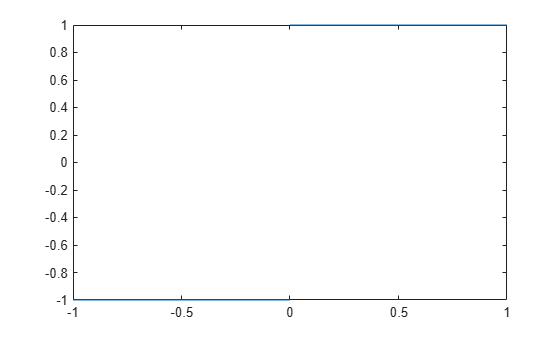Documentation

### This is machine translation

Mouseover text to see original. Click the button below to return to the English version of the page.

Note: This page has been translated by MathWorks. Click here to see
To view all translated materials including this page, select Country from the country navigator on the bottom of this page.

## Limits

The fundamental idea in calculus is to make calculations on functions as a variable “gets close to” or approaches a certain value. Recall that the definition of the derivative is given by a limit

`$f\text{'}\left(x\right)=\underset{h\to 0}{\mathrm{lim}}\frac{f\left(x+h\right)-f\left(x\right)}{h},$`

provided this limit exists. Symbolic Math Toolbox™ software enables you to calculate the limits of functions directly. The commands

```syms h n x limit((cos(x+h) - cos(x))/h, h, 0)```

which return

```ans = -sin(x)```

and

`limit((1 + x/n)^n, n, inf)`

which returns

```ans = exp(x)```

illustrate two of the most important limits in mathematics: the derivative (in this case of cos(x)) and the exponential function.

### One-Sided Limits

You can also calculate one-sided limits with Symbolic Math Toolbox software. For example, you can calculate the limit of x/|x|, whose graph is shown in the following figure, as x approaches 0 from the left or from the right.

```syms x fplot(x/abs(x), [-1 1], 'ShowPoles', 'off')```To calculate the limit as x approaches 0 from the left,

`$\underset{x\to {0}^{-}}{\mathrm{lim}}\frac{x}{|x|},$`

enter

```syms x limit(x/abs(x), x, 0, 'left')```
```ans = -1```

To calculate the limit as x approaches 0 from the right,

`$\underset{x\to {0}^{+}}{\mathrm{lim}}\frac{x}{|x|}=1,$`

enter

```syms x limit(x/abs(x), x, 0, 'right')```
```ans = 1```

Since the limit from the left does not equal the limit from the right, the two- sided limit does not exist. In the case of undefined limits, MATLAB® returns `NaN` (not a number). For example,

```syms x limit(x/abs(x), x, 0)```

returns

```ans = NaN```

Observe that the default case, `limit(f)` is the same as `limit(f,x,0)`. Explore the options for the `limit` command in this table, where `f` is a function of the symbolic object `x`.

Mathematical Operation

MATLAB Command

$\underset{x\to 0}{\mathrm{lim}}f\left(x\right)$

`limit(f)`

$\underset{x\to a}{\mathrm{lim}}f\left(x\right)$

`limit(f, x, a) or`

`limit(f, a)`

$\underset{x\to a-}{\mathrm{lim}}f\left(x\right)$

`limit(f, x, a, 'left')`

$\underset{x\to a+}{\mathrm{lim}}f\left(x\right)$

`limit(f, x, a, 'right')`

#### Mathematical Modeling with Symbolic Math Toolbox

Get examples and videos